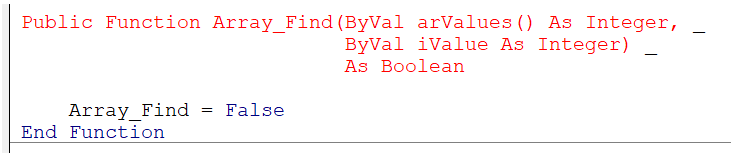# User FAQs

If you have a question, please send it to us.

1) How would you declare a Fixed Array of Integers ?
This array contains 11 items: arValues(0) to arValues(10).

`Dim arValues1(10) As Integer Dim arValues2(0 To 10) As Integer Debug.Print LBound(arValues1) & "-" & UBound(arValues1)  '0-10  Debug.Print LBound(arValues2) & "-" & UBound(arValues2)  '0-10  `

2) How would you declare a Dynamic Array of Integers ?

`Dim arValues() As Integer `

3) What is the difference between a Fixed Array and a Dynamic Array ?
A dynamic array can be resized multiple times at run-time using the ReDim statement.
A fixed array cannot be resized and the size is defined when it is declared.

4) What is the difference between the following two lines of code ?

`Dim arValues() As Variant Dim arValues As Variant `

The first line declares an array of the data type Variant.
The second line declares a Variant data type which can hold any data type (including an array).
Variant Data Type vs Array of Variants.

5) Is it possible to resize a Dynamic Array ?
Yes. You can use the ReDim statement.
This can only change the size of the upper bound (not the lower bound).
You can only change the size of the last dimension if the array has multiple dimensions.

`Dim arValues() As Integer ReDim arValues(2) arValues(1) = 10 arValues(2) = 20 ReDim arValues(6) `

6) Is it possible to resize a Dynamic Array without losing the current values ?
Yes. You can use the keyword Preserve.

`ReDim Preserve arValues(6) `

7) How would you initialise an Array of Integers ?
You can either initialise one by one or inside a loop.

`Dim arValues(3) As Integer arValues(0) = 10 arValues(1) = 20 arValues(2) = 30 arValues(3) = 40 `

8) Is it possible to use the ARRAY function to initialise an array ?
Yes. The Array must be declared with a Variant data type.
ARRAY - Returns an array containing the given values.

`Dim arValues() As Variant arValues = VBA.Array(10,20,30,40) `

9) Is it possible to initialise an Array without looping ?
Yes. This code will initialise the array to contain the value 8.
In Excel VBA you can use a combination of the Evaluate method and the IF and ISERROR functions.

`Dim arValues(3) As Integer arValues = Application.Evaluate("IF(ISERROR(A1:D1), 8, 8)") `

10) What function would you use to quickly filter an array of values based on a substring ?
FILTER - Returns an array containing a subset of values that contain a substring (Variant).
The filtered array contains all the items that contain the letter "e".

`Dim arValues(2) As String Dim arFiltered As Variant arValues(0) = "mon" arValues(1) = "tue" arValues(2) = "wed" arFiltered = VBA.Filter(arValues, "e") 'arFiltered(0) = "tue"'arFiltered(1) = "wed"`

11) Is it possible to start an array at index position 1 (instead of 0) ?
Yes. There are two ways this can be done.
You can explicitly define the base of the array.

`Option Base 0  'default  Dim arValues2(1 To 10) As Integer Debug.Print LBound(arValues2) & "-" & UBound(arValues2)  '1-10  `

You can place Option Base 1 at the top of a module.
The Option Base statement only affects the module it is declared in.

`Option Base 1 Dim arValues2(10) As Integer Debug.Print LBound(arValues2) & "-" & UBound(arValues2)  '1-10  `

12) How can you tell if an Array is 0 or 1 based ?
You can use the LBOUND function to return the lower bound of the array.

`If (VBA.LBound(arValues) = 0) Then If (VBA.LBound(arValues) = 1) Then `

13) How many items are there in this Fixed Array ?

`Option Base 0 Dim arValues(3) As Integer `

There are four items: arValues(0), arValues(1), arValues(2) and arValues(3).

14) How many items are there in this Fixed Array ?

`Option Base 1 Dim arValues(3) As Integer `

There are three items: arValues(1), arValues(2) and arValues(3).

15) How would you declare a 2-Dimensional Fixed Array ?

`Dim arValues(1 to 10, 1 to 4) As String `

16) How would you declare a 2-Dimensional Dynamic Array ?

`Dim arValues( , ) As String `

17) What would the syntax be for passing in a Fixed Array into a subroutine ?

`Public Sub MySubroutine(ByRef arValues() As String) Public Sub MySubroutine(ByRef arValues As Variant) Public Sub MySubroutine(ByVal arValues As Variant) `

18) Is there any difference between the following two lines of code ?

`Public Sub MySubroutine(ByRef arValues() As Integer) Public Sub MySubroutine(ByVal arValues() As Integer)   'compile error  `

The first line will pass in an array by reference.
The second line will generate a compile error because arrays cannot be passed by value. They must always be passed by reference.

19) What would the syntax be for returning a Fixed Array from a function ?

`Public Function MyFunction() As String() Public Function MyFunction() As Long() Public Function MyFunction() As Variant `

20) What is wrong with the following Function declaration ?When you pass in an array, you must pass the "arValues" as ByRef and not ByVal.

© 2022 Better Solutions Limited